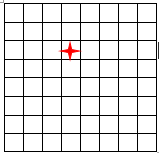# Count The Squares

Probability Level 4

Consider the following $8\times8$ grid. Count the total number of squares that do not contain the square with the red star.×